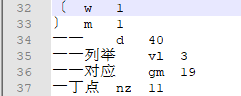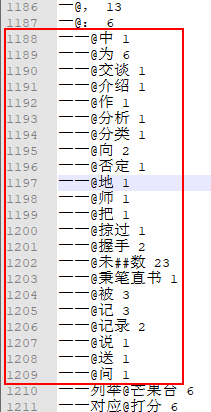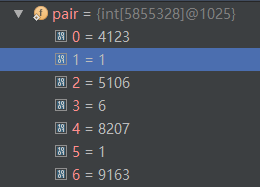# HanLP二元核心词典详细解析

ObjectInputStream in = new ObjectInputStream(IOUtil.newInputStream(path));

while ((line = br.readLine()) != null){

String[] params = line.split("\\s");

String[] twoWord = params.split("@", 2);

...

}

TreeMap<Integer, TreeMap<Integer, Integer>> map = new TreeMap<Integer, TreeMap<Integer, Integer>>();

int idA = CoreDictionary.trie.exactMatchSearch(a);//二元接续的 @ 前的内容

int idB = CoreDictionary.trie.exactMatchSearch(b);//@ 后的内容

TreeMap<Integer, Integer> biMap = map.get(idA);

if (biMap == null){

biMap = new TreeMap<Integer, Integer>();

map.put(idA, biMap);//

}

biMap.put(idB, freq);

map中保存二元核心词典示意图如下：/**

* 描述了词在pair中的范围，具体说来<br>

* 给定一个词idA，从pair[start[idA]]开始的start[idA + 1] - start[idA]描述了一些接续的频次

*/

static int start[];//支持快速地二分查找

/**

* pair[偶数n]表示key，pair[n+1]表示frequency

*/

static int pair[];

start 数组

int maxWordId = CoreDictionary.trie.size();

...

start = new int[maxWordId + 1];

for (int i = 0; i < maxWordId; ++i){

TreeMap<Integer, Integer> bMap = map.get(i);

if (bMap != null){

for (Map.Entry<Integer, Integer> entry : bMap.entrySet()){

//省略其他代码

++offset;//统计以 这个词 为前缀的所有二阶共现的个数

}

}//end if

start[i + 1] = offset;

}// end outer for loop

if (bMap != null)表示 第 i 个词(i从下标0开始)在二元词典中有二阶共现，于是 统计以 这个词 为前缀的所有二阶共现的个数，将之保存到 start 数组中。下面来具体举例，start数组中前37个词的值如下：pair 数组

pair数组的长度是二元核心词典行数的两倍

int total = 0;

while ((line = br.readLine()) != null){

//省略其他代码

total += 2;

}

pair数组 偶数 下标 存储 保存的是 一元核心词典中的词 的下标，而对应的偶数加1 处的下标 存储 这个词的共现频率。即： pair[偶数n]表示key，pair[n+1]表示frequency

pair = new int[total];  // total是接续的个数*2

for (int i = 0; i < maxWordId; ++i)

{

TreeMap<Integer, Integer> bMap = map.get(i);//i==0?

if (bMap != null)//某个词在一元核心词典中, 但是并没有出现在二元核心词典中(这个词没有二元核心词共现)

{

for (Map.Entry<Integer, Integer> entry : bMap.entrySet())

{

int index = offset << 1;

pair[index] = entry.getKey();//词 在一元核心词典中的id

pair[index + 1] = entry.getValue();//频率

}

}

}

'一 一'在 map 中第0号位置处， 它是一元核心词典中的第 34个词。   共有 22个共现词。如下：0=4123 （‘中’在一元核心词典中的位置（从下标0开始计算））

1=1 （'一 一@中'的词共现频率）

2=5106 （'为' 在一元核心词典中的位置） 【为 p 65723】

3=6 ('一 一@为'的词共现频率)@中 1

@为 6

@交谈 1

@介绍 1

@作 1

@分析

.......//省略其他

public static int getBiFrequency(int idA, int idB){

//省略其他代码

int index = binarySearch(pair, start[idA], start[idA + 1] - start[idA], idB);

return pair[index + 1];

}

start 数组保存了一元词典中每个词 在二元词典中的词共现情况： start[idA] 代表 idA在 pair 数组中共现词的起始位置，而start[idA + 1] - start[idA]代表 以idA 为前缀的共现词一共有多少个，这样二分查找的范围就只在 start[idA] 和 start[idA] + (start[idA + 1] - start[idA]) - 1之间了。

private static int binarySearch(int[] a, int fromIndex, int length, int key)

{

int low = fromIndex;

int high = fromIndex + length - 1;

//省略其他代码

//只列出关键代码

List<Vertex> vertexList = viterbi(wordNetAll);//求解词网的最短路径

to.updateFrom(node);//更新权重

double weight = from.weight + MathTools.calculateWeight(from, this);//计算两个顶点(idA->idB)的权重

int nTwoWordsFreq = CoreBiGramTableDictionary.getBiFrequency(from.wordID, to.wordID);//查核心二元词典

int index = binarySearch(pair, start[idA], start[idA + 1] - start[idA], idB);//二分查找 idA@idB共现频率

• 博文量
172
• 访问量
131109